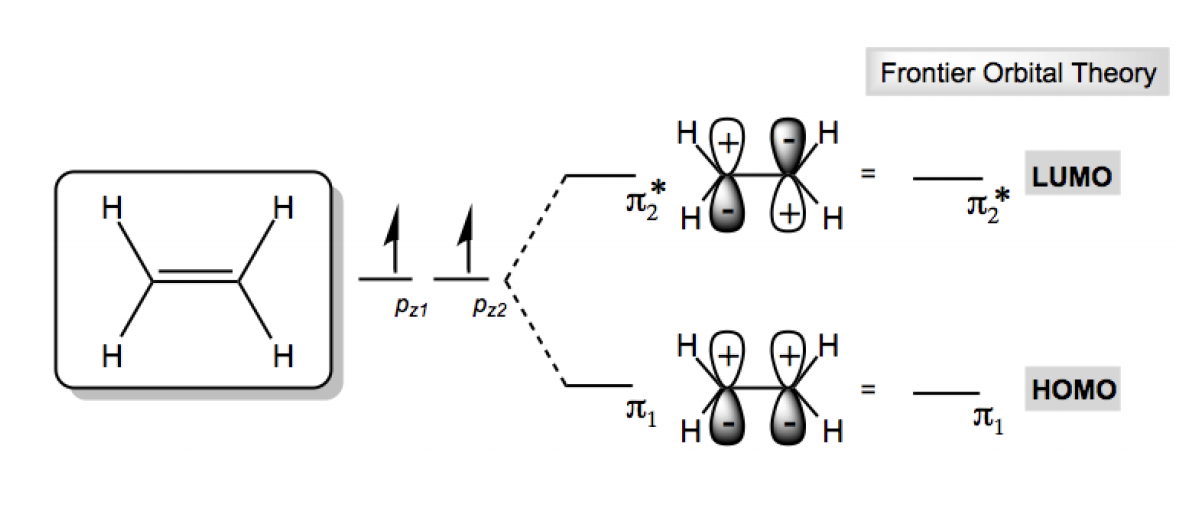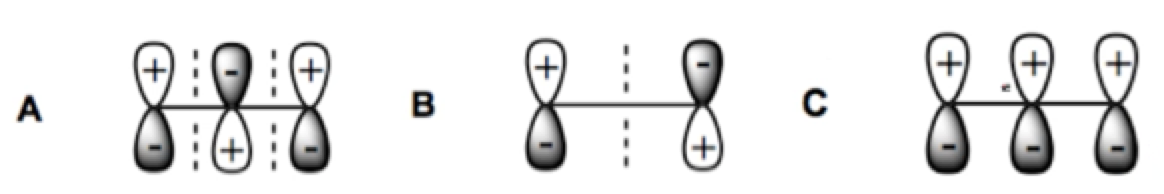Clutch Prep is now a part of Pearson
Ch. 16 - Conjugated SystemsWorksheetSee all chapters

# HOMO LUMO

See all sections
Sections
Conjugation Chemistry
Stability of Conjugated Intermediates
Allylic Halogenation
Conjugated Hydrohalogenation (1,2 vs 1,4 addition)
Diels-Alder Reaction
Diels-Alder Forming Bridged Products
Diels-Alder Retrosynthesis
Molecular Orbital Theory
Drawing Atomic Orbitals
Drawing Molecular Orbitals
HOMO LUMO
Orbital Diagram: 3-atoms- Allylic Ions
Orbital Diagram: 5-atoms- Allylic Ions
Orbital Diagram: 6-atoms- 1,3,5-hexatriene
Orbital Diagram: Excited States
Pericyclic Reaction
Thermal Electrocyclic Reactions
Photochemical Electrocyclic Reactions
Cumulative Electrocyclic Problems
Sigmatropic Rearrangement
Cope Rearrangement
Claisen Rearrangement
Diene

Frontier orbital interations are the driving force behind many Organic Chemistry recations. Let's find out why.

Concept #1: HOMO vs. LUMO

Transcript

Hey everyone. In this video I want to introduce a very important concept in organic chemistry called frontier molecular orbital theory, let's take a look, frontier orbital interactions are actually the riding force behind many reactions in organic chemistry, so this information that we're going to learn now it's actually going to be foundational to understand reactions later, so it's really important that we know what this FMOT theory is, okay? And the most important thing that we need to learn about frontier molecular orbitals is how to find HOMO and LUMO, so you might have heard about HOMO and LUMO before but in this lesson we really need to fully understand it, so the HOMO is defined as the highest occupied molecular orbital, so out of all your molecular orbitals it's the one that has the highest energy that still has electrons in it, okay? then there's the LUMO, the LUMO is the lowest unoccupied molecular orbital, so once again, out of all you molecular orbitals, it's the one that has the lower energy while still having no electrons in it, okay? So, if we were to look at ethene as an example, so ethene is a very simple molecule so it's easy to understand HOMO, LUMO.

So, what we would have is we have two atomic orbitals, right? And those two atomic orbitals form two molecular orbitals, we have the bonding orbital, we have the antibonding orbital, and according to the principles of electron configuration, this two electrons should only fill the bottom pi 1 orbital, it's called pi 1, not zi 1, because it's just a double bond, you just call it pi 1, and that means pi 2 star, which is an antibonding orbital is unfilled, okay? So what that means is that our HOMO orbital is pi 1, so we would say that the HOMO contains two electrons, it's pi 1 and that means that my LUMO orbital is pi 2 and has zero electrons, that's it, so HOMO is the highest one that still has electrons, which in this case it's the only one, because it's the only one that exists and LUMO has no electrons in it and it's the lower energy state, but since there's only two it just happens to be the only one that doesn't have electrons, as you molecules get bigger and the number of molecular orbitals increases, finding HOMO, LUMO will be on that, basically the cusp of where the electrons end and where the unfilled orbitals begin, so let's go ahead and try out a practice problem.

• HOMO = Highest Occupied Molecular Orbital
• LUMO = Lowest Unoccupied Molecular OrbitalPractice: Consider the MO's of allyl anion. Which of the following are HOMO and LUMO?